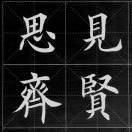V1

2022/05/15阅读：18主题：红绯

# Tikz作图教程：图论中环的画法

Tikz作图教程：图论中环的画法

## 一、对命令的解释

### 1. \Vertex

\Vertex[<options>]{<name>}
• <options>里常用选项有坐标, Math，例如\Vertex[Math,x=1,y=0](x)的意思是设置顶点x的坐标为(1,0), 顶点标签用数学字体显示。如果省略坐标，则默认该点位于(0,0)，即坐标原点。

• <name>是这个顶点的名称，定义了名为x的顶点以后，下面就可以用(x)来引用它。

### 2. \Edge

\Edge[<options>](<vertex1>)(<vertex2>)
• 一条边有两个顶点，所以，需要说明它的两个顶点。
• 中括号里是对边的风格进行说明。常见的设置有：颜色、虚实、标签。例如 [red,dashed,label={$e_1$}]意为这条边为“红色，虚线，标签为 ”。

### 3. \Loop

\Loop[<options>]{<vertex>}
• 环的风格除了具有与边的类似的那些选项以外，还有有大小、朝向等等独有的选项。例如, [dist=2cm,dir=NO,style={-,dashed,red},label={$e_4$}], 其中，dist=2cm控制环的大小。dir=NO表示环的方向朝北（与地图方位一样，表示在顶点的上方）。类似的，dir=SO朝下，dir=WEdir=EA分别表示朝左和朝右。

## 二、例子

### 1.边的标签位于边上

\begin{tikzpicture}[edge1/.style={red,dashed,bend left},edge2/.style={blue, bend left},] \tikzset{LabelStyle/.style = {shape=rectangle,draw=none,fill=brown!50,font=\normalsize,text =black}}  \Vertex[Math]{y}  \Vertex[Math,x=0,y=3]{x}  \Edge[style=edge2,label={$e_3$}](x)(y)  \Edge[style=edge1,label={$e_2$}](y)(x)  \Loop[dist=2cm,dir=NO,style={-,dashed,red},label={$e_4$}](x)  \Loop[dist=2cm,dir=SO,style={-,dashed,red},label={$e_1$}](y)\end{tikzpicture}

### 2.边的标签位于边的附近

\begin{tikzpicture}[auto,every node/.style={shape=circle,draw},edge1/.style={red,dashed,bend left},edge2/.style={blue, bend left},]\tikzset{LabelStyle/.style = {draw=none,font=\normalsize,text =black}}
• tikzpicture环境后中括号内增加选项auto;
• 边的标签设置改为：draw=none，即不画出标签边框，不填充。

### 3.将点的风格设置为art

\begin{tikzpicture}[auto,every node/.style={shape=circle,draw},edge1/.style={red,dashed,bend left},edge2/.style={blue, bend left},]\GraphInit[vstyle=art]\tikzset{LabelStyle/.style = {draw=none,font=\normalsize,text =black}}

## 三、练习V1